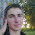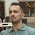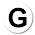VHDL coding tips and tricks: Sequence detector using state machine in VHDL

## Sunday, October 31, 2010

### Sequence detector using state machine in VHDL

Some readers were asking for more examples related with state machine and some where asking for codes related with sequence detector.This article will be helpful for state machine designers and for people who try to implement sequence detector circuit in VHDL.
I have created a state machine for non-overlapping detection of a pattern "1011" in a sequence of bits. The state machine diagram is given below for your reference.

The VHDL code for the same is given below. I have added comments for your easy understanding.

library IEEE;
use IEEE.STD_LOGIC_1164.ALL;

--Sequence detector for detecting the sequence "1011".
--Non overlapping type.
entity seq_det is
port(   clk : in std_logic;  --clock signal
reset : in std_logic;   --reset signal
seq : in std_logic;    --serial bit sequence
det_vld : out std_logic  --A '1' indicates the pattern "1011" is detected in the sequence.
);
end seq_det;

architecture Behavioral of seq_det is

type state_type is (A,B,C,D);  --Defines the type for states in the state machine
signal state : state_type := A;  --Declare the signal with the corresponding state type.

begin

process(clk)
begin
if( reset = '1' ) then     --resets state and output signal when reset is asserted.
det_vld <= '0';
state <= A;
elsif ( rising_edge(clk) ) then   --calculates the next state based on current state and input bit.
case state is
when A =>   --when the current state is A.
det_vld <= '0';
if ( seq = '0' ) then
state <= A;
else
state <= B;
end if;
when B =>   --when the current state is B.
if ( seq = '0' ) then
state <= C;
else
state <= B;
end if;
when C =>   --when the current state is C.
if ( seq = '0' ) then
state <= A;
else
state <= D;
end if;
when D =>   --when the current state is D.
if ( seq = '0' ) then
state <= C;
else
state <= A;
det_vld <= '1';   --Output is asserted when the pattern "1011" is found in the sequence.
end if;
when others =>
NULL;
end case;
end if;
end process;

end Behavioral;

If you check the code you can see that in each state we go to the next state depending on the current value of inputs.So this is a mealy type state machine.
The testbench code used for testing the design is given below.It sends a sequence of bits "1101110101" to the module. The code doesnt exploit all the possible input sequences. If you want another sequence to be checked then edit the testbench code.  If it is not working as expected, let me know.

LIBRARY ieee;
USE ieee.std_logic_1164.ALL;

ENTITY blog_cg IS
END blog_cg;

ARCHITECTURE behavior OF blog_cg IS

signal clk,reset,seq,det_vld : std_logic := '0';
constant clk_period : time := 10 ns;

BEGIN

-- Instantiate the Unit Under Test (UUT)
uut: entity work.seq_det PORT MAP (
clk => clk,
reset => reset,
seq => seq,
det_vld => det_vld
);

-- Clock process definitions
clk_process :process
begin
clk <= '0';
wait for clk_period/2;
clk <= '1';
wait for clk_period/2;
end process;

-- Stimulus process : Apply the bits in the sequence one by one.
stim_proc: process
begin
seq <= '1';             --1
wait for clk_period;
seq <= '1';             --11
wait for clk_period;
seq <= '0';             --110
wait for clk_period;
seq <= '1';             --1101
wait for clk_period;
seq <= '1';             --11011
wait for clk_period;
seq <= '1';             --110111
wait for clk_period;
seq <= '0';             --1101110
wait for clk_period;
seq <= '1';             --11011101
wait for clk_period;
seq <= '0';             --110111010
wait for clk_period;
seq <= '1';             --1101110101
wait for clk_period;
wait;
end process;

END;

The simulated waveform is shown below:

Note:- The code was simulated using Xilinx 12.1 version. The results may vary slightly depending on your simulation tool.

#### 24 comments:

1.In process(clk) - reset is missing from the sensitivity list.

2.@foam : thanks for the note. As you said, it should be process(clk,reset).

3.What would be the state diagram for an overlapping sequence? What is the difference?

4.@karan : Read any digital book for the state diagram for overlapping sequence detector.
For converting the state diagram into a vhdl code, you can use the same concept used in this post.

1.Which book is good for vhdl

5.How to draw the internal architecture of 4 bit non overlapping sequence detector????

6.When should I use the state machine design used in this example and when should I use the design used in http://vhdlguru.blogspot.com/2010/04/how-to-implement-state-machines-in-vhdl.html?

It seems to me that in this example you change the current state in the same clock as it's verified, but in the other one you let it wait to change in the next clock. Is my interpretation correct?

7.@Leandro : Really good question and observation. You can use this type of design in all cases.
See the sensitivity list in both the processes. In this design it has Clk.So in each clk edge it will check and change the state.
But in the second code, the sensitivity list contains only next state and input. Which means that if they remain the same for some reason(depends on your state machine design) then the process statemnent may not evaluate in every clock cycle.

8.@sonica : I think you can find the answer in any good digital design book. The above example deals with a non overlapping sequence. So draw the state machine in a similar way.Once you have the state machine(which is the most important step) coding it in vhdl is a very easy process.

9.Hai
I have project in vhdl to be completed within next week.The project is to decode irig b pulses.pls help me in doing that

mahesh

10.i want to ask...when i run the testbench and this error come out : Error (10533): VHDL Wait Statement error at blog_cg.vhd(26): Wait Statement must contain condition clause with UNTIL keyword

11.want sequence detector for 0100 and 1000 sequences

12.please send me the state diagram with the necessary explanation for the below Question.on my email id (the.beast.master.007@gmail.com)
A sequential network has on input (X) and two outputs (Z1 and Z2). An output Z1=1 occurs every time the input sequence 010 is completed provided that the sequence 100 has never occurred.an output Z2=1 occurs every time the iniput sequence 100 is completed. Note that once a Z2=1 output has occurred, Z1=1 can never occur,but not vice versa.
a).Derive a mealy state graph and table with a minimum number of states (8 states).

13.@beast_boy : Contact me using the contact form and we will discuss about it.

14.in the test bench i think is a mistake.... it didn't show the state signal... can you help me to fix the problem... i try to do something but the signal state in simulation is block on state A... some help someone?

15.@sabin : contact me using the contact form. I can help for a fee.

1.hi i have the same problem, can`t see state change, the state is always A ..
can you help me, please ?

16.Hi, the program you have mentioned above seems to me helpful to detect ADS-S signal(https://docs.google.com/document/d/1EzR8wpxjFJhmnaxeSt7_6DNqb-bFPda8emZF6W1kIhI/edit?pli=1) for my project.
All I need to detect only pattern of the signal (only 10 microsecond from the beginning not the entire 120 microsecond in attached figure).

As I am very new to VHDL and so difficult to proceed.
Can I get some more Idea how I can proceed to do simulation to detect this signal?

Thanks in Advance
Sheikh

17.thank u bt in simulation we are not getting 'state' ;

18.Hello to everybody . Please tell me what i must do for having "state" in TEST BENCH SIMULATION ??? Thanks ...

1.I am eager to see the answer. I want to to see that too.

19.if it is a overlapping sequence in the last state D after encountering '1' it must go to state B RIGHT?Please correct me if I am wrong

20.What if I wanted to detect this sequence five times

21.Can you please send the code for moore sequence detector for sequence-0111010